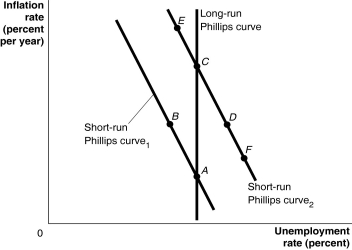# [Solved] Figure 1311 Alt Text for Figure 13

## Figure 13.11Alt text for Figure 13.11: In figure 13.11, a graph shows the short-run and long-run Phillips curves.Long description for Figure 13.11: The x-axis is labelled, unemployment rate percent, and the y-axis is labelled, inflation rate percent per year.A straight line labelled, short-run Philips Curve 1, begins at the top left corner and slopes down to the bottom center.A straight line labelled, short-run Philips Curve 2, follows the same slope as Curve 1, but is plotted to the right.A straight line labelled, long-run Philips Curve, is perpendicular to the x-axis, and intersects the short-run Philips Curve 1 at point A near the bottom end of both lines.Point B is plotted half way along the short-run Philips Curve 1.Long-run Philips Curve intersects short-run Philips Curve 2 at point C near the top half of the curves.Point D is plotted more than half way along the short-run Philips Curve 2.Points E and F are plotted close to the left and right ends, respectively, of the short-run Philips Curve 2.-Refer to Figure 13.11.A(n)________ would be depicted as a movement from A to D to C.

A)supply shock, such as rising oil prices,
B)increase in aggregate demand
C)implementation of contractionary monetary policy
D)increase in short-run aggregate supply
E)expansionary fiscal policy

Tags
Choose question tag10+ million students use Quizplus to study and prepare for their homework, quizzes and exams through 20m+ questions in 300k quizzes.

Explore our library and get Economics Homework Help with various study sets and a huge amount of quizzes and questions

### Related Questions

Get Free Access Now!